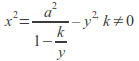## Examples of Fluid Flows

 Name of Flow Velocity Function Complex Potential Equation of Streamlines Diagram Uniform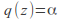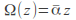If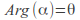then streamlines have equation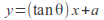where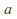is an arbitrary constant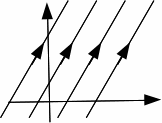Flow With Stagnation Point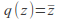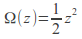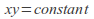Source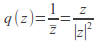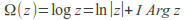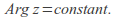Streamlines radiate out from the origin, following straight lines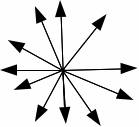Vortex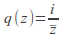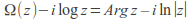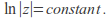This equation implies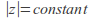and is the equation of a circle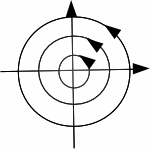Doublet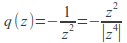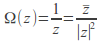Either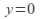or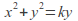or equivalently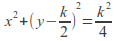A Doublet in a Uniform Stream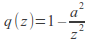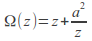Either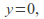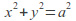or